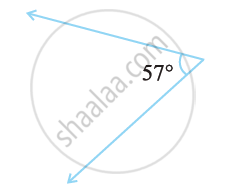# Find the Complement of the Following Angles: -3 - Mathematics

Find the complement of the following angles:#### Solution

The sum of the measures of complementary angles is 90º.

57°

Complement = 90° − 57°

= 33°

Concept: Related Angles - Complementary Angles
Is there an error in this question or solution?

#### APPEARS IN

NCERT Class 7 Maths
Chapter 5 Lines and Angles
Exercise 5.1 | Q 1.3 | Page 101